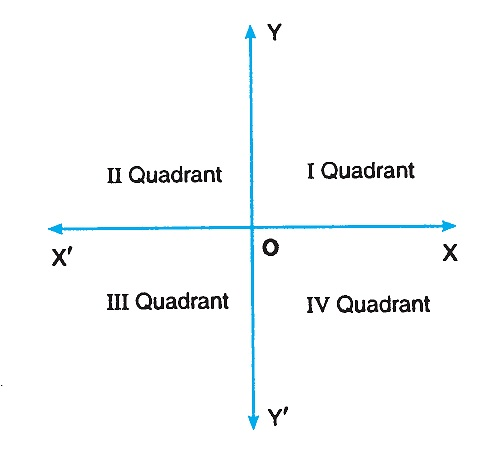# Coordinate System

The position of a point in a plane is fixed w.r.t. to its distances from two axes of reference, which are usually drawn by the two graduated number lines XOX′ and YOY′, at right angles to each other at O.

The horizontal number line XOX′ is called x-axis and the vertical number line YOY′ is called y-axis. The point O, where both axes intersect each other is called the origin. The two axes together are called rectangular coordinate system.The positive direction of x-axis is taken to the right of the origin O, OX and the negative direction is taken to the left of the origin O, i.e., the side OX′. Similarly, the portion of y-axis above the origin O, i.e., the side OY is taken as positive and the portion below the origin O, i.e., the side OY′ is taken as negative.